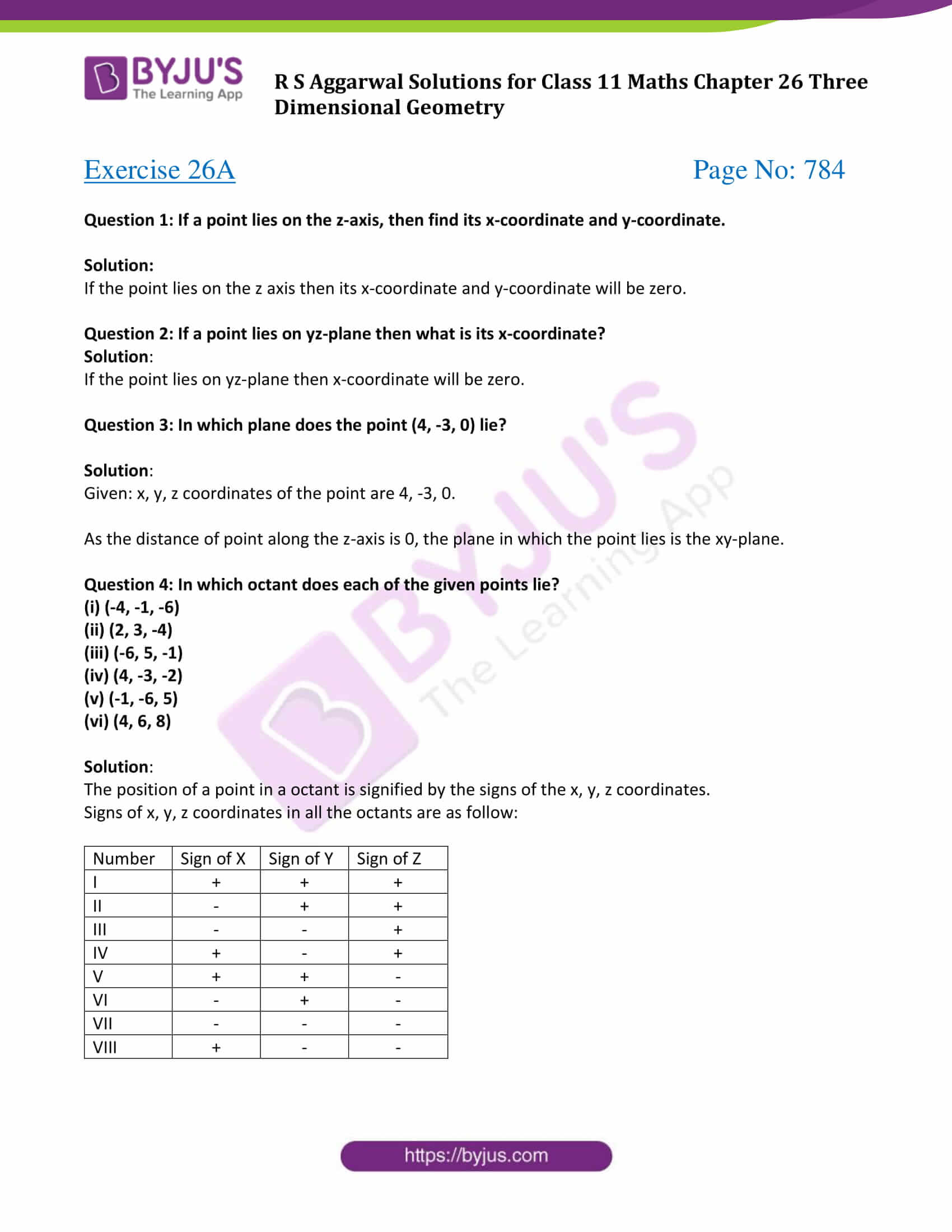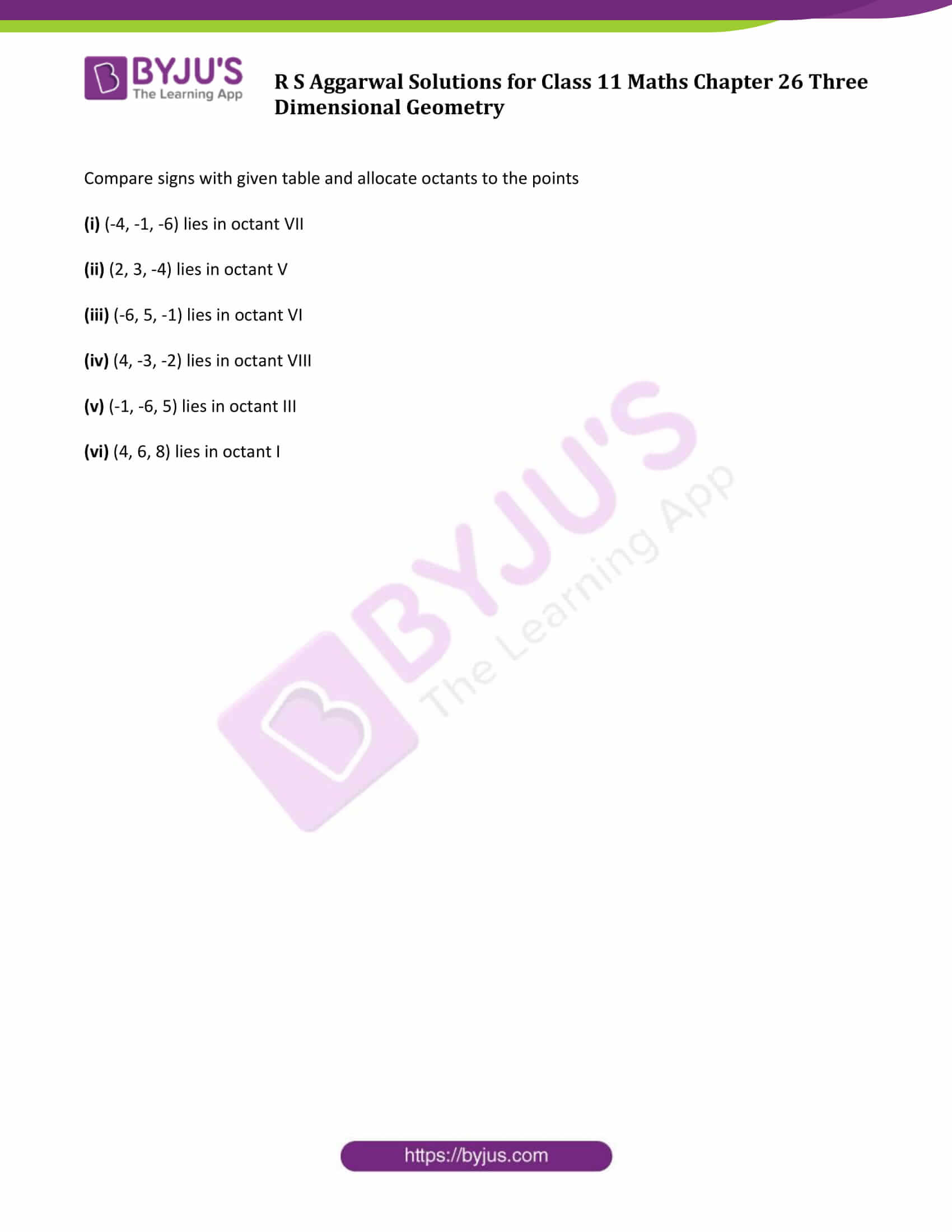# R S Aggarwal Solutions for Class 11 Maths Chapter 26 Three Dimensional Geometry Exercise 26A

R S Aggarwal Solutions for Class 11 Maths are given here for Chapter 26 exercise 26A. The set of questions and answers that we are offering in RS Aggarwal Solutions have been carefully prepared for class 11 students by subject experts. This exercise is based on the location of a point in three dimensional coordinate system. Check the detailed R S Aggarwal solutions for class 11 chapters and start practicing to score good marks.

## Download PDF of R S Aggarwal Solutions for Class 11 Maths Chapter 26 Three Dimensional Geometry Exercise 26A### Access Answers of R S Aggarwal Solutions for Class 11 Maths Chapter 26 Three Dimensional Geometry Exercise 26A Page number 784

Exercise 26A Page No: 784

Question 1: If a point lies on the z-axis, then find its x-coordinate and y-coordinate.

Solution:

If the point lies on the z axis then its x-coordinate and y-coordinate will be zero.

Question 2: If a point lies on yz-plane then what is its x-coordinate?

Solution:

If the point lies on yz-plane then x-coordinate will be zero.

Question 3: In which plane does the point (4, -3, 0) lie?

Solution:

Given: x, y, z coordinates of the point are 4, -3, 0.

As the distance of point along the z-axis is 0, the plane in which the point lies is the xy-plane.

Question 4: In which octant does each of the given points lie?

(i) (-4, -1, -6)

(ii) (2, 3, -4)

(iii) (-6, 5, -1)

(iv) (4, -3, -2)

(v) (-1, -6, 5)

(vi) (4, 6, 8)

Solution:

The position of a point in a octant is signified by the signs of the x, y, z coordinates.

Signs of x, y, z coordinates in all the octants are as follow:

 Number Sign of X Sign of Y Sign of Z I + + + II – + + III – – + IV + – + V + + – VI – + – VII – – – VIII + – –

Compare signs with given table and allocate octants to the points

(i) (-4, -1, -6) lies in octant VII

(ii) (2, 3, -4) lies in octant V

(iii) (-6, 5, -1) lies in octant VI

(iv) (4, -3, -2) lies in octant VIII

(v) (-1, -6, 5) lies in octant III

(vi) (4, 6, 8) lies in octant I

## Access other exercise solutions of Class 11 Maths Chapter 26 Three Dimensional Geometry

Exercise 26B Solutions

Exercise 26C Solutions

## R S Aggarwal Solutions for Class 11 Maths Chapter 26 Exercise 26A

Class 11 Maths Chapter 26 Three Dimensional Geometry Exercise 26A is based on the topic – Coordinates of a point in space. In this exercise, students have to find the location of the points in space.